# 卡尔曼滤波 -- 从推导到应用(二)

28 篇文章 47 订阅

clc
clear all
close all

% 初始化参数
delta_t=0.1;   %采样时间
t=0:delta_t:5;
N = length(t); % 序列的长度
sz = [2,N];    % 信号需开辟的内存空间大小  2行*N列  2:为状态向量的维数n
g=10;          %加速度值
x=1/2*g*t.^2;      %实际真实位置
z = x + sqrt(10).*randn(1,N); % 测量时加入测量白噪声

Q =[0 0;0 9e-1]; %假设建立的模型  噪声方差叠加在速度上 大小为n*n方阵 n=状态向量的维数
R = 10;    % 位置测量方差估计，可以改变它来看不同效果  m*m      m=z(i)的维数

A=[1 delta_t;0 1];  % n*n
B=[1/2*delta_t^2;delta_t];
H=[1,0];            % m*n

n=size(Q);  %n为一个1*2的向量  Q为方阵
m=size(R);

% 分配空间
xhat=zeros(sz);       % x的后验估计
P=zeros(n);           % 后验方差估计  n*n
xhatminus=zeros(sz);  % x的先验估计
Pminus=zeros(n);      % n*n
K=zeros(n(1),m(1));   % Kalman增益  n*m
I=eye(n);

% 估计的初始值都为默认的0，即P=[0 0;0 0],xhat=0
for k = 9:N           %假设车子已经运动9个delta_T了，我们才开始估计
% 时间更新过程
xhatminus(:,k) = A*xhat(:,k-1)+B*g;
Pminus= A*P*A'+Q;

% 测量更新过程
K = Pminus*H'*inv( H*Pminus*H'+R );
xhat(:,k) = xhatminus(:,k)+K*(z(k)-H*xhatminus(:,k));
P = (I-K*H)*Pminus;
end

figure
plot(t,z);
hold on
plot(t,xhat(1,:),'r-')
plot(t,x(1,:),'g-');
legend('含有噪声的测量', '后验估计', '真值');
xlabel('Iteration');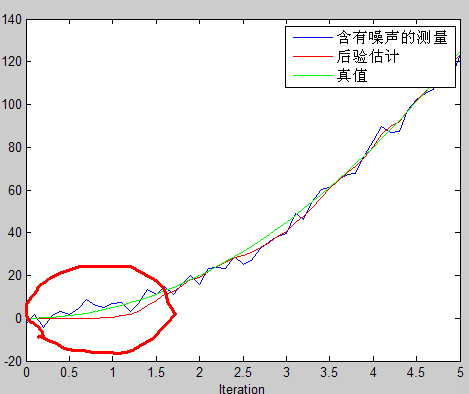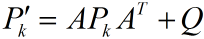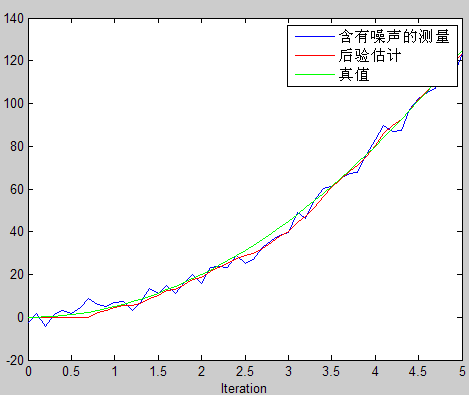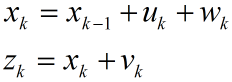clc
clear
close all

% 初始化参数
delta_t=0.1;
t=0:delta_t:5;
g=10;%加速度值
n_iter = length(t); % 序列的长度
sz = [n_iter, 1]; % 信号需开辟的内存空间大小
x=1/2*g*t.^2;
x=x';
z = x + sqrt(10).*randn(sz); % 测量时加入测量白噪声

Q = 0.9; % 过程激励噪声方差
%注意Q值得改变  待会增大到2，看看效果。对比看效果时，修改代码不要改变z的值
R = 10; % 测量方差估计，可以改变它来看不同效果

% 分配空间
xhat=zeros(sz);      % x的后验估计
P=zeros(sz);         % 后验方差估计
xhatminus=zeros(sz); % x的先验估计
Pminus=zeros(sz);    % 先验方差估计
K=zeros(sz);         % Kalman增益

% 估计的初始值
xhat(1) = 0.0;
P = 1.0;
for k = 2:n_iter   %
% 时间更新过程
xhatminus(k) = xhat(k-1);
Pminus(k) = P(k-1)+Q;

% 测量更新过程
K(k) = Pminus(k)/( Pminus(k)+R );
xhat(k) = xhatminus(k)+K(k)*(z(k)-xhatminus(k));
P(k) = (1-K(k))*Pminus(k);
end

figure
plot(t,z);
hold on
plot(t,xhat,'r-')
plot(t,x,'g-');
legend('含有噪声的测量', '后验估计', '真值');
xlabel('Iteration');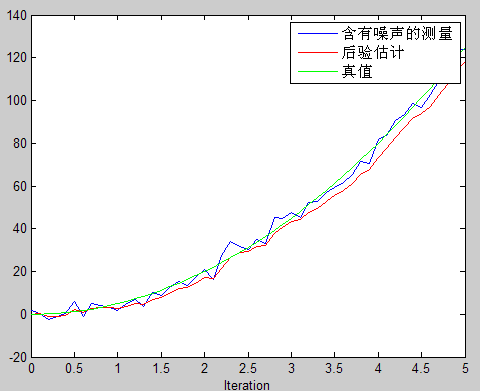图(a)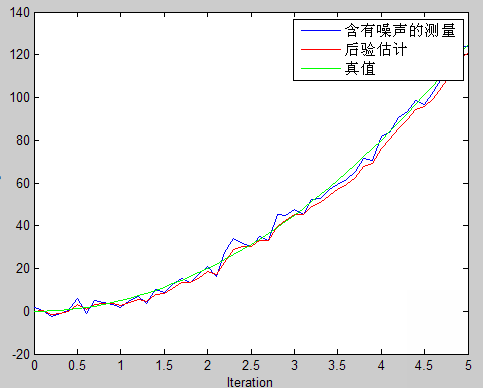图(b)

全文完

04-1610-21
09-08
09-275万+
02-10808
04-065839
04-21
12-031万+
12-3020万+
09-24202
11-131039
11-011万+
01-11
05-044万+
03-07312
03-318936
05-127283
02-119万+点击重新获取扫码支付余额充值